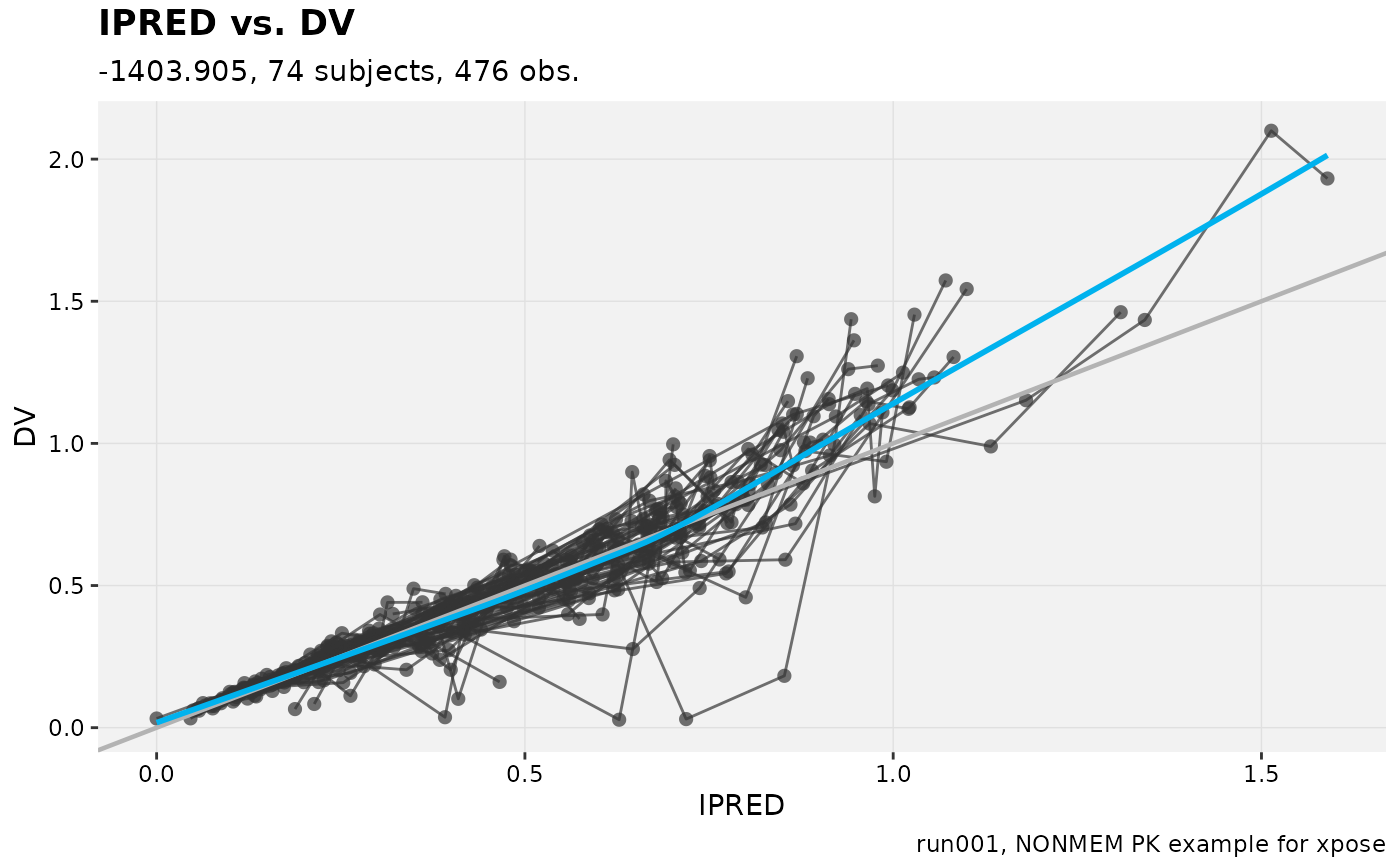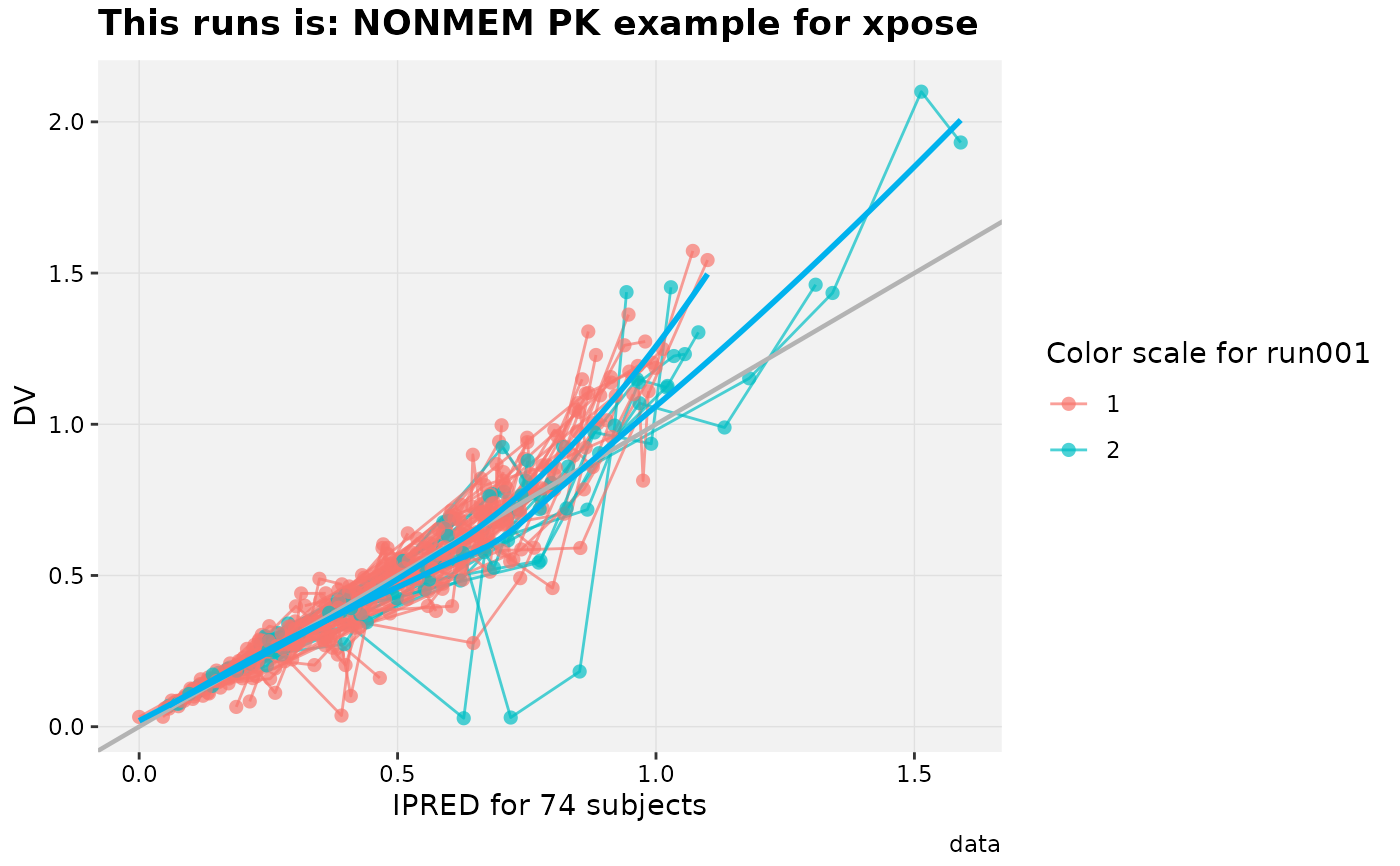Template titles can be used to create highly informative diagnostics plots. They can be applied to any plot title, subtitle, caption and the filename when saving with the xpose_save function.

Template titles are defined via a single string containing key variables staring with a @ (e.g. @ofv) which will be replaced by their actual value when rendering the plot. For example '@run, @nobs observations in @nind subjects' would become 'run001, 1022 observations in 74 subjects'

Many key variables are available:

@condn

Condition number

@covtime

Covariance matrix runtime

@data

Model input data used

@descr

Model description

@dir

Model directory

@epsshk

Epsilon shrinkage

@errors

Run errors (e.g termination error)

@esampleseed

ESAMPLE seed number (used in NPDE)

@etashk

Eta shrinkage

@file

Model file name

@label

Model label

@method

Estimation method or sim

@nesample

Number of ESAMPLE (used in NPDE)

@nind

Number of individuals

@nobs

Number of observations

@nsig

Number of significant digits

@nsim

Number of simulations

@ofv

Objective function value

@page and @lastpage

Are respectively the page number and the number of the last page when faceting on multiple pages

@probn

Problem number

@plotfun

Name of the plot function

@ref

Reference model

@run

Model run name

@runtime

Estimation/Sim runtime

@software

Software used (e.g. NONMEM)

@simseed

Simulation seed

@subroutine

Differential equation solver

@timestart

Run start time

@timestop

Run stop time

@timeplot

Time of the plot rendering

@term

Termination message

@version

Software version (e.g. 7.3)

@vpcci

VPC confidence interval

@vpcdir

VPC data directory

@vpclloq

VPC lower limit of quantification

@vpcnsim

Number of simulations for VPC

@vpcpi

VPC prediction interval

@vpculoq

VPC upper limit of quantification

@warnings

Run warnings (e.g. boundary)

@x @y etc.

Name of any ggplot2 variable used for mapping in an aes() type function

# Defined when creating a plot
caption = '@run, @descr')#> Using data from $prob no.1#> Filtering data by EVID == 0#> geom_smooth() using formula 'y ~ x'#> geom_smooth() using formula 'y ~ x'# Any label can be modified later on dv_vs_ipred(xpdb_ex_pk, aes(point_color = SEX, line_color = SEX)) + labs(title = 'This runs is: @descr', color = 'Color scale for @run', x = 'IPRED for @nind subjects', subtitle = NULL)#> Using data from$prob no.1#> Filtering data by EVID == 0#> geom_smooth() using formula 'y ~ x'#> geom_smooth() using formula 'y ~ x'